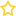## Mathematics

Sort by:

##### College Exam Math Prep
This Course is FREE

### Course Overview

The Acellus College Exam Math Preparation course has been specially developed to help students prepare to take the math portion of the ACT or SAT college entrance exam. Students watch as problems similar to...

### College Exam Math Prep(0 Votes)

#### This Course is FREE

18469 Viewers
##### AP Calculus AB Exam Prep
This Course is FREE

### Course Overview

The AP Calculus AB Exam Prep course was designed for students to quickly and efficiently review many concepts typically covered on the AP Calculus AB Exam. After being shown step-by-step how to solve several...

### AP Calculus AB Exam Prep(0 Votes)

#### This Course is FREE

5482 Viewers
##### Pre-Calculus
This Course is FREE

### Course Overview

Acellus Pre-Calculus covers topics that students must understand to tackle the rigorous Acellus AP Calculus AB course. Course topics include:
• Functions
• Parallel and Perpendicular Lines
• Analytic Trigonometry
• Vectors, Parametric, and Polar
• Systems and Matrices
• Conics
• ...

### Pre-Calculus(0 Votes)

#### This Course is FREE

21855 Viewers
##### Trigonometry
This Course is FREE

### Course Overview

In Acellus Trigonometry, students learn about the relationships between the sides and the angles of triangles and how to make calculations based on them using trigonometric functions.  Acellus Trigonometry is taught by award-winning Acellus...

### Trigonometry(0 Votes)

#### This Course is FREE

17763 Viewers
##### Integrated Mathematics III
This Course is FREE

### Course Overview

Acellus Integrated Mathematics III, taught by Patrick Mara, is the last course of a three-part series that includes algebra, geometry, probability, and statistics. This high school math pathway is patterned after an approach typically...

### Integrated Mathematics III(0 Votes)

#### This Course is FREE

11902 Viewers
##### Integrated Mathematics II
This Course is FREE

### Course Overview

Acellus Integrated Mathematics II, taught by Patrick Mara, is the second course of a three-part series that includes algebra, geometry, probability, and statistics. This high school math pathway is patterned after an approach typically...

### Integrated Mathematics II(0 Votes)

#### This Course is FREE

9916 Viewers
##### Integrated Mathematics I
This Course is FREE

### Course Overview

Acellus Integrated Mathematics I, taught by Patrick Mara, is the first course of a three-part series that includes algebra, geometry, probability, and statistics. This high school math pathway is patterned after an approach typically...

### Integrated Mathematics I(0 Votes)

21935 Viewers
Page 3 of 3123